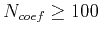Time-to-depth conversion and seismic velocity estimation using time-migration velocityNext: Examples Up: Inversion Methods Previous: Finite difference method

## Spectral Chebyshev method

Alternatively, we solve our PDE in the form given by equation 15 by a spectral Chebyshev method Boyd (2001). Using cubic splines, we define the input data atChebyshev points. We compute the Chebyshev coefficients and the coefficients of the derivatives in the right-hand side of equation 15. Then we use a smaller numberof the coefficients for function evaluation. We need to do such Chebyshev differentiation twice. Finally we perform the time step using the stable third-order Adams-Bashforth method Boyd (2001), which is(23)

whereis the right-hand side. In numerical examples, we triedand. This method allows larger time steps than the finite difference, and has the adjustable parameter.

For step 2, we use a Dijkstra-like solver introduced in Cameron et al. (2007). It is an efficient time-to-depth conversion algorithm motivated by the Fast Marching Method (Sethian, 1996). The input for this algorithm isand the outputs are the seismic velocityand the transition matrices from time-domain to depth-domain coordinatesand. We solve the eikonal equation with an unknown right-hand side coupled with the orthogonality relation(24)

The orthogonality relation means that the image rays are orthogonal to the wavefronts. Such time-to-depth conversion is very fast and produces the outputs directly on the depth-domain grid.Time-to-depth conversion and seismic velocity estimation using time-migration velocityNext: Examples Up: Inversion Methods Previous: Finite difference method

2013-03-02